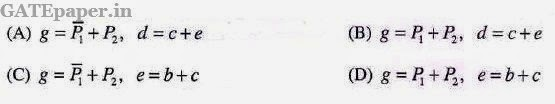### GATE 2009 ECE Video Solution on Digital Circuits (Digital Electronics)

1. In a microprocessor, the service routine for a certain interrupt starts from a fixed location of memory which cannot be externally set, but the interrupt can be delayed or rejected. Such an interrupt is

2. If X = 1 in the given logic equation, thena. Y = Z
b. Y = Z’
c. Z = 1
d. Z = 0

3. What are the minimum number of 2 to 1 multiplexers required to generated a 2 input AND gate and a 2 input EX-OR gate?
a. 1 and 2
b. 1 and 3
c. 1 and 1
d. 2 and 2

4. Refer to the NAND and NOR latches shown in the figure. The inputs (P1, P2) for both the latches are first made (0, 1) and then, after a few seconds, made (1, 1). The corresponding stable outputs (Q1, Q2) are5. What are the counting stages (Q1, Q2) for the counter shown in the figure below?a. 11, 10, 00, 11, 10….
b. 01, 10, 11, 00, 01….
c. 00, 11, 01, 10, 00…..
d. 01, 10, 00, 01, 10…..

Two products are sold from a vending machine, which has two push buttons P1 and P2. When a button is pressed, the price of the corresponding product is displayed in a 7-segment display.
If no buttons are pressed, ‘0’ is displayed, signifying ‘Rs. 0’.
If only P1 is pressed, ‘2’ is displayed, signifying ‘Rs. 2’.
If only P2 is pressed, ‘5’ is displayed, signifying ‘Rs. 5’.
If both P1 and P2 are pressed, ‘E’ is displayed, signifying ‘Error’.
The names of the segments in the 7-segment display, and the glow of the display for ‘0’, ‘2’, ‘5’ and ‘E’ are shown below.Consider
(i) Push button pressed / not pressed is equivalent to logic 1 / 0 respectively
(ii) A segment glowing / not glowing in the display is equivalent to logic 1 / 0 respectively
6. If segments a to g are considered as functions of P1 and P2, then which of the following is correct?7. What are the minimum numbers of NOT gates and 2-inout OR gates required to design the logic of the driver for this 7-segment display?
a. 3 NOT and 4 OR
b. 2 NOT and 4 OR
c. 1 NOT and 3 OR
d. 2 NOT and 3 OR
Solution (6 & 7) : https://www.youtube.com/watch?v=njEr-LoSbw4